# Denissa

Denissa has 18 9/10 kilos of bananas to sell if she was able to sell 15 ⅗. How many kilos of bananas were left?

x =  3.3 kg

### Step-by-step explanation: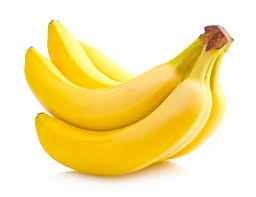Did you find an error or inaccuracy? Feel free to write us. Thank you!## Related math problems and questions:

• How many 15How many six-eights are there in 18? Which of the following would you choose to solve this question? 18 x 6/8 or 18 : 6/8
• To improper fractionChange mixed number to improper fraction a) 1 2/15 b) -2 15/17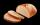They brought in d two kilos and t three kilos of bread to the store. By the end of the day, 14 two-kg bread were sold. How many kilos of bread remained in the store by the next day?
• Speed of Slovakian trainsRudolf decided to take the train from the station 'Ostratice' to 'Horné Ozorovce'. In the train timetables found train Os 5409 : km 0 Chynorany 15:17 5 Ostratice 15:23 15:23 8 Rybany 15:27 15:27 10 Dolné Naštice 15:31 15:31 14 Bánovce nad Bebravou 15:35 1
• The fish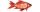The fish vendor sells 1/7 kilos of bangus for 66.2 euro. If you will buy 9 2/8 kilos of bangus, how much will cost?
• PoolIf water flows into the pool by two inlets, fill the whole for 19 hours. The first inlet filled pool 5 hour longer than the second. How long pool take to fill with two inlets separately?
• A ropeA rope can be cut into equal length with no rope left over. The lengths can be 15cm,18cm or 25cm. What is the shortest possible length of the rope?
• TheatreThe theatrical performance started at 15:50 and ended at 18:50. How many minutes long?
• A burger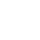The water starts with 41 1/4 cups of water how much water is left after ten 3 5/8 Scoops of water are removed?
• The sum 12The sum of 3 mixed numbers is 20 13/15. two of the numbers are 6 1/3 and 7 5/6. what is the third number?
• Two pizzasJacobs mom bought two whole pizzas. He ate 2/10 of the pizza and his dad ate 1 1/5. How much is left.
• Sum of 20Sum of the two numbers is 15 1/6. If one of the numbers is 2 2/3, find the other number.
• Brother and sisterWhen I was 8, my sister was 4, and now I am 18. How old is my sister?
• Dividing walnuts into crates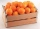There are 8 and 2 over 3 pounds of walnuts in a container, which will be divided equally into containers that hold 1 and 1 over 5 pounds. This would fill n and 4 over 18 containers. What is n?
• Kilos of meat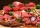Marry bought 6 1/3 kg of meat. She used 2 1/4 kg for Anita 2 1/8 kg for Maros and the rest for Adam. How many kilograms of meat did she used for Adam?
• Bananas plantation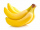Ashok Rao planted bananas on 2/7 th of his field on his 21 acers. What is the area of the bananas plantation?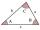The size of two internal angles of a triangle ABC are α=6/18π and β=7/18π. Calculate the size of the third angle.